Interview First Aid
Starter's Guide to Case Prep
Crack the Case Interview
Ace the Personal Fit Interview
Master the Problem Solving Test
Case Cracking

# Apply these shortcuts to impress your interviewer with outstanding mental math skills

The best way to prepare for the quantitative portion of case interviews is to practice the types of calculations you will need to perform with numbers representative of actual Case Numbers and to be familiar with the types of problems you are most likely to encounter and how to solve them. As a reminder, candidates cannot use calculators or spreadsheets in the case interviews but may use pen and paper. All the Case Calculations take this into account, so Case Numbers and Case Calculations usually have certain characteristics you can use to simplify the calculations (if you are familiar with certain calculation methods).

For example, Case Numbers are usually round numbers with only a few significant digits, such as 300,000 or 4,000,000. While some people may be intimidated by having to do calculations with large numbers like this without a calculator, once you learn how to efficiently manage the zeroes, calculations with numbers like these become straightforward.

When Case Numbers have more than one significant digit, they usually have additional properties that can make certain calculations like multiplication and division much easier if you are aware of the appropriate methods. For example, let’s say you are told a company sells a product for \$32 per unit and sells 25,000 units per year, and you are asked to calculate the revenue. You would then need to do the calculation: (\$32 × 25,000). Most people would use pen and paper for this calculation. You can make the pen-and-paper calculation more efficient by not writing out the three zeroes from “25,000” and then adding them to the end of the result of (32 × 25).

These numbers are representative of actual Case Numbers, and here is an efficient method for this calculation (32 × 25). First decompose 25 as: (25 = ¼ × 100). Then we perform the calculation using this decomposition of 25.

32 × ¼ = 32 ÷ 4 = 8

8 × 100 = 800

Therefore (32 × 25 = 800) - you can check this with a calculator or pen-and-paper calculation. You can think of this new calculation method as follows: let’s say you are given 32 quarters (U.S. coins); how much money would you have? You might think to yourself: “Well, 4 quarters make 1 dollar, and 32 ÷ 4 = 8, so I would have \$8,” which is correct. Now think about how many cents are in \$8. The answer is clearly 800: (8 × 100 = 800), as there are 100 cents in 1 dollar. So 32 quarters make \$8, which is 800 cents. Now a quarter is also worth 25 cents, and since we have 32 quarters, then 32 × 25 = 800. So to multiply any number by 25, you divide the number by 4 and then multiply by 100. This method works because (4 × 25 = 100); so, when you divide 32 by 4, the result is the “number of groups of 100.” Now the answer to our original problem of (\$32 × 25,000) is then \$800,000.

Using this method relies on the fact that 25 = ¼ × 100. These numbers are representative of actual Case Numbers, and most actual multiplication calculations in case interviews involve numbers like 25,000 or 25 Million, for which there is a way to dramatically simplify the calculation.

Many case interview preparation resources have example calculations that you are highly unlikely to encounter in an actual case interview, such as multiplying by a number like 23,487, which has five significant digits and no clear way to simplify the calculation. Practicing quantitative problems that are not representative of actual Case Numbers of Case Calculations is not an effective way to prepare, as you won’t be practicing for what you will actually face in an interview. If the practice problems involve numbers with many significant digits, this becomes over-kill as you just practice brute-force mechanical calculations, and don’t practice the logical problem-solving aspects, and don’t get experience looking for and finding efficient calculation methods.

## Effective Quantitative Preparation Resources

This article has numerous examples of the calculation methods taught in the FastMath Ace the Case online course and includes efficient solution methods to the example problems given in the previous article.

## Mental Math Skills Are Extremely Valuable in Case Interviews

Because you cannot use calculators in case interviews — and because you may need to perform a large number of math operations — being able to do calculations mentally (without relying on pen and paper for the mechanics of calculations) is a very helpful skill in case interviews.

Consider the following example, where you are given Price, Quantity and Profit Margin for three different products and asked to calculate the percentage of the firm’s overall revenue and profit contributed by each product: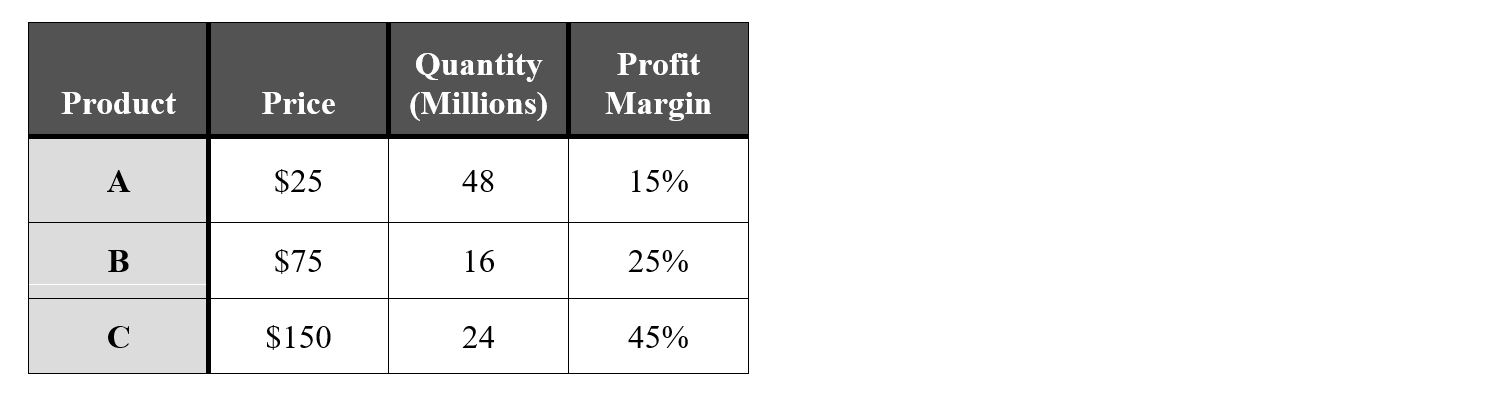Let’s say your approach to answering this question is to first calculate the revenue and profit for each product. If you can do these operations mentally (and write down the answers) rather than writing out all the multiplication operations in longhand, you will have a large advantage in calculation speed. Longhand multiplication refers to doing a multiplication calculation like 7 × 25 as shown below: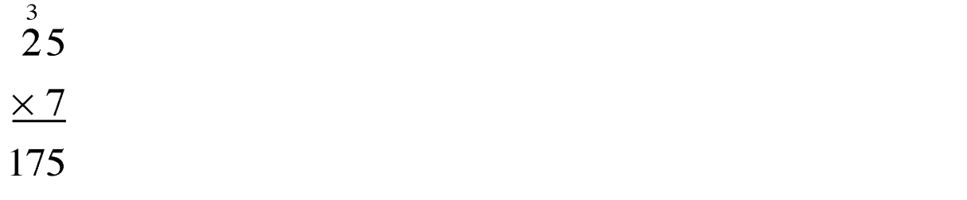If you need to do each multiplication operation from the above problem in longhand format, you would first need to copy the multiplication terms from the table onto pen and paper, and then do the calculation. Since calculating the revenue and profit for each product requires a large number of multiplication operations, a person who needs to do each multiplication operation in longhand format will be much slower than a person who can do the calculations mentally. It is much faster and more efficient to simply do each calculation mentally (without writing the original numbers out in longhand format) and then write down the result. The speed improvement of mental calculations is magnified if you need to multiply several numbers together when calculating a value.

For example: Profit = (Price) × (Quantity) × (Profit Margin)

For mental calculations, it is very helpful to “mentally picture” the numbers with which you are calculating —which I may refer to as “thinking” of a certain number or numbers. After doing a calculation mentally, you should always write down the result so you don’t forget it.

Given that the Case Numbers have few significant digits—and often have trailing significant digits of “5,” “25” or “75”—performing addition and subtraction with Case Numbers is relatively straightforward for most people. You may want to practice adding and subtracting numbers if you are rusty or don’t feel confident in being able to do this smoothly in an interview, which has added stress.

Example: Add the following numbers: 250 Million, 300 Million, and 150 Million.

### Methods for adding large numbers:

A helpful method for adding large numbers is to calculate with Units like Thousand (K), Million (M), Billion (B), and Trillion (T):

Units

K: Thousand

M: Million

B: Billion

T: Trillion

When adding large numbers, don’t write out (or mentally picture the number with) all the zeroes; simply write (or mentally picture) the leading digits and an abbreviation for the unit. For example, to represent “250 Million”—which, in long-form, would be written as “250,000,000”—write/think “250 M,” or mentally remember the Unit and do the calculations and, when you write down the result, include the appropriate Unit.

In case interviews, if you are given printed sheets with numerical values or tables of numbers, the printout will usually not include all the zeroes for a number like 25 Million; it will use a Unit like “Million” or the table will indicate the values are in “Millions” without writing out all the zeroes.

Using these Units, the prior example becomes: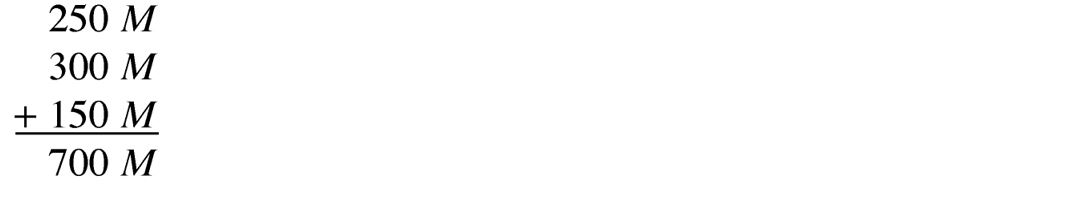## Multiplication methods

Multiplication is the most important quant skill to practice for case interviews because you will frequently have to perform numerous multiplication operations - sometimes involving three or more terms. You can improve your speed and efficiency with multiplication while reducing errors by learning and practicing a few efficient calculation methods. Below are a few examples of how to apply efficient calculation methods to case interview multiplication calculations.

Example: Calculate 160 × 350 M

Writing this calculation out in longhand with all the zeroes is a slow, error-prone process, as many people will make a mistake with the number of zeroes. It is also difficult to do this calculation mentally while keeping track of all the zeroes, and people frequently make a similar error regarding the number of zeroes with a mental calculation, which causes the answer to be off by a factor of 10 or more. This is clearly a very bad mistake to make in a job interview.

FastMath Solution Method

The FastMath Solution Method is different from how most people would approach this, but each step is very simple:

Step 1: What’s 160 ÷ 2

Answer: 160 ÷ 2 = 80

Step 2: What’s 350 × 2

Answer: 350 × 2 = 700

Step 3: What’s 80 × 700

Answer: 80 × 700 = 56,000

Guess what? That’s the same answer as 160 × 350 — you can check it with a calculator!

We get the answer by cutting 160 in half, which is 80, and doubling 350, which is 700, and multiplying these results (80 × 700). This is called the Halve and Double Method.

So the answer to the original problem is 56,000 Million, which is 56 Billion.

### Bullet Operator for Multiplication

To improve the readability of equations, we may use the “bullet” (also called the “dot”) symbol “⋅” to indicate multiplication. Therefore, the equation (20 × 4 = 80) could be written as (20 ⋅ 4 = 80) with the bullet/dot symbol. This can improve the readability of equations where you are multiplying the number of terms as the dot symbol is smaller than the cross symbol “×” and so it’s easier to read variable names and numbers.

Example: Calculate 125 ⋅ 2.5 ⋅ 4

Another method useful for multiplying numbers is to reorder the multiplication operations into a different sequence that is easier to calculate. We can multiply these numbers in this sequence:

125 ⋅ 4 = 500

500 ⋅ 2.5 = 1,250

We’ll call this the “Reordering” method, and it uses the mathematical property that, when multiplying a set of numbers, the order in which you multiply terms doesn’t matter; we’ll call this property the Reordering Property of multiplication. There are many potential ways in which you can Reorder the multiplication terms, and you can choose the order that is easiest for the particular numbers involved. There may be multiple efficient/effective ways to Reorder multiplication terms, and there is no single “correct” sequence. We could also calculate this as follows:

2.5 ⋅ 4 = 10

125 ⋅ 10 = 1,250

Most people find these new orders are much easier to execute than multiplying the first pair of numbers in the original calculation (125 ⋅ 2.5).

These are a few efficient multiplication methods covered—you can preview a video lesson that explains these and other multiplication methods in further detail here: Multiplication Lesson

## Division Methods

Some Case Calculations require division, and you frequently need to express the result as a percentage. There is usually a way to simplify the division Case Calculations, so you frequently don’t need to use Long Division. Candidates should still be familiar with and be prepared to use Long Division if they cannot find a simplification method, and candidates may encounter some Case Calculations where traditional Long Division is appropriate.

Below are a few examples of how to apply efficient calculation methods to division Case Calculations.

Example: Calculate 42 Billion ÷ 500

Doing the calculation using Long Division and writing out all the zeroes is a slow, error-prone process. It is also difficult to do this calculation purely mentally because of all the zeroes.

First, we write 42 Billion using our units, which becomes 42 B.

Second, we treat 500 as: (500 = ½ ⋅ 1,000 = ½ ⋅ K)

Then we calculate using this “decomposition” of 500.

42 B ÷ K = 42 M (since B ÷ K = M)

Next, we need to divide by ½, which is the same as multiplying by 2.

42 M ⋅ 2 = 84 M

To divide by 1,000 (K), we simply do the inverse of multiplying by 1,000 (K) and decrease the Unit size. Hence: (B ÷ K = M). We could do these operations in any order, so we could multiply by 2 first, and then divide by K.

## Multiplying and Dividing with “Clean” Numbers

Many of the Case Numbers involved in multiplication and/or division calculations in case interviews will have special properties and are what we will call “Clean Numbers,” defined as being a “Clean Fraction” times an Integer power of 10. In this context, a “Clean Fraction” means both the numerator and denominator (what we will call the “elements” of the fraction) are non-zero integers that are each smaller than 10 - and often smaller than 5.

For example, the number 25 can be represented as ¼ ⋅ 100; hence, 25 is a Clean Number, as the elements of the fraction are “1” and “4”, which are both smaller than 5. Below are some examples of how to express certain numbers as Clean Fractions times an Integer power of 10, showing that they are Clean Numbers: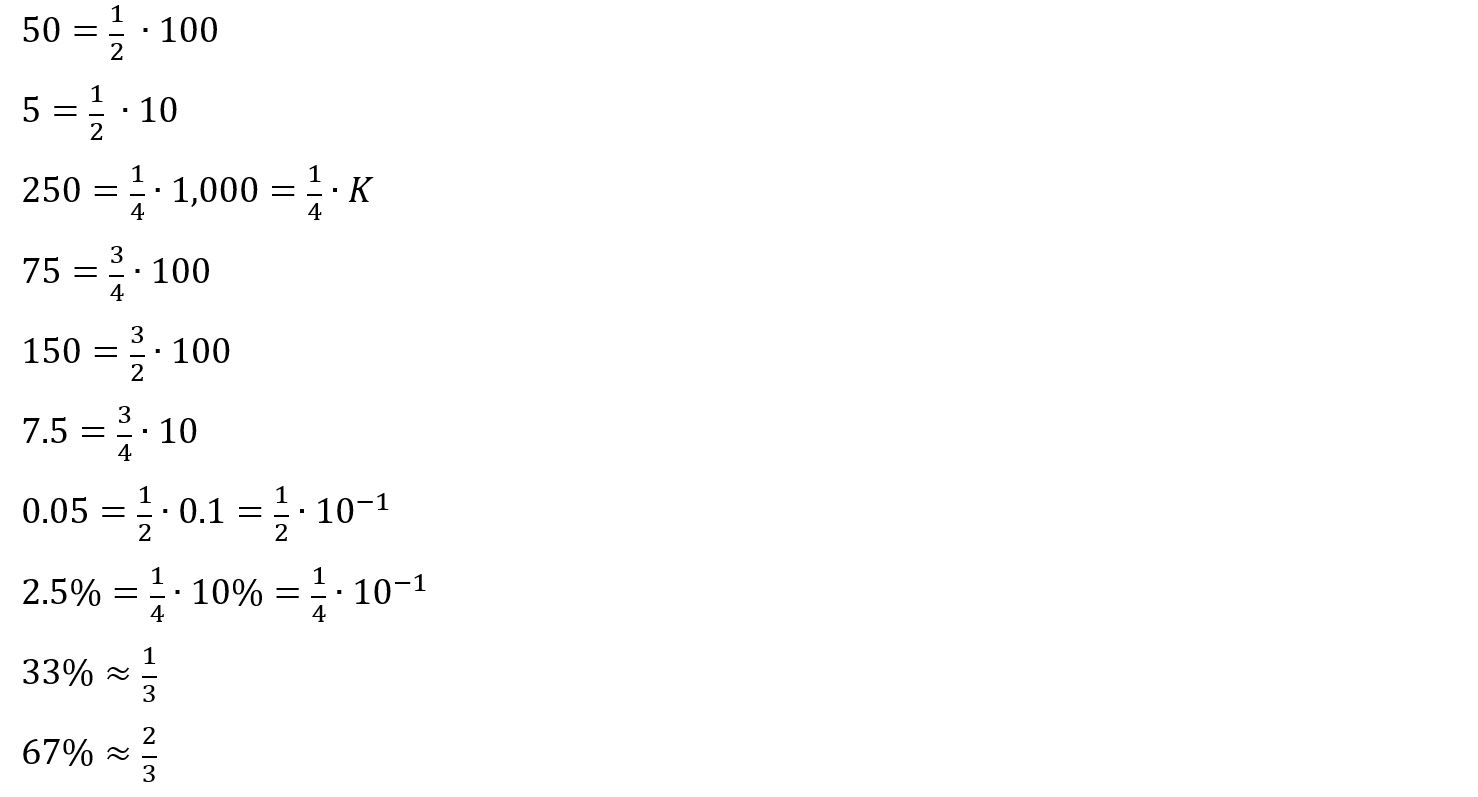A few things to note about Clean Numbers:

• The Numerator can be larger than 1, as shown with 75 = ¾ ⋅ 100.
• The Numerator can be larger than the Denominator, as shown with 150.
• The Integer exponent of 10 can be positive or negative, as shown with 0.05—which has a negative exponent of 10.
• You can represent percentages as a Clean Fraction, as shown with 2.5%.
• 33% and 67% are approximately equal to ⅓ and ⅔, respectively (the symbol “≈” means “approximately equal to.” These fractions are actually repeating decimals, but, in Case Interviews, you can often round these percentage values to these Clean Fractions.

There are specific efficient calculation methods you can learn and apply to make multiplying and dividing with Clean Numbers much easier than traditional calculation methods. The key concept of these efficient calculation methods is to identify Clean Numbers and multiply/divide with them while expressing them as a Clean Fraction times a power of 10. Keep in mind that, when multiplying two numbers, only one number needs to be a Clean Number to use these efficient calculation methods. With the example of 250 ⋅ 120 M, only 250 is a Clean Number.

## Percentage Growth Methods

Example: A company had annual revenue of \$400 Million in the past calendar year, and their revenue is projected to grow at 4% per year. Approximately, what will the company’s revenue be six years in the future?

The most efficient way to approximate the answer is to ignore compounding, which we will call the “non-compounding approximation”. Since the revenue grows 4% per year, for six years, if we ignore compounding, total revenue would grow by (4% ⋅ 6 = 24%). Since compounding will contribute slightly more growth, it is appropriate to round the total growth to 25%, which is ¼. Since the original revenue is \$400 M, the net change in revenue is 25% ⋅ \$400 M = \$100 M. Therefore, the revenue in six years is \$500 M = (\$400 M + \$100 M).

It is appropriate to ignore compounding when the percentage change and the number of years of growth are relatively small. The FastMath Ace the Case online course explains in further detail when you can use the non-compounding approximation and provides additional methods for performing percentage-growth calculations when it’s not appropriate to use this approximation.

## Net Present Value (NPV) Methods

NPV Example 1: How much would your company be willing to pay for another company that generates \$20 Million in profit annually, if your firm requires an annual return on investment of 10%?

There is a formula to determine the net NPV for an annuity, which is a fixed payment that occurs annually (\$C), for a given required annual return or interest rate (r), which is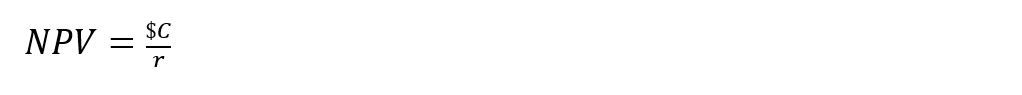Given that r =10%,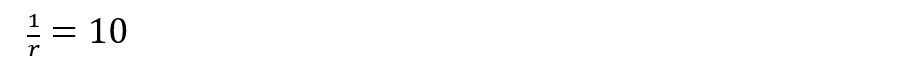Therefore, NPV = 10 ⋅ \$C = 10 ⋅ \$20 M = \$200 M.

Your company would therefore be willing to pay up to \$200 Million to acquire this firm.

NPV Example 2: A real estate development firm is evaluating a project that involves buying a parcel of land and building condominiums on that parcel. The company forecasts they can sell the condominiums for a total of \$250 Million six years in the future.

What is the maximum the real estate company would be willing to spend now to buy the land and develop the condominiums, if all the associated costs for the project would be incurred today, and they require a 12% annual return on invested capital?

This is a trickier NPV calculation than the prior one, as this is not an annuity, and we have to determine the NPV of a payment of \$250 M in six years. There is a concept called the Rule of 72, which tells us that something growing at 12% annually will double in approximately six years, as: (6 ⋅ 12 = 72). In general, the doubling time for a quantity will be approximately 72 divided by the percentage growth rate (expressed as an integer) - in this example, 72 ÷ 12 = 6. This approximation is accurate for percentage growth in the range of 0%–20%.

Therefore, the NPV of a payment of \$250 M in six years, with a required return of 12%, is exactly half the future payment value of \$250 M, which is \$125 M.

The FastMath Ace the Case online course has an in-depth lesson on the Rule of 72, which explains when and how to use it, and why the approximation is accurate.

You can learn more about and register for this online course through the URL below, which applies a ~20% discount to the original price of \$179.

Registration URL

The FastMath Ace the Case online course provides a comprehensive set of tools to prepare for the quantitative portion of Case Interviews. This online course uses video-based instruction to teach efficient calculation methods to improve your speed and efficiency for the most common Case Calculations (multiplication, division, percentages, and compound growth calculations). The methods in this course are specialized for typical Case Numbers, and all the examples in this online course are based on real Case Calculations. The video lessons in this course demonstrate how to apply these efficient calculation methods to the most common types of quantitative Case Interview problems, such as Market Sizing problems and Break-even Analysis problems, and show how to solve these types of problems most efficiently. This course also includes practice problems and solution methods that reinforce the course material.

Matthew Tambiah is a former McKinsey consultant and has a Bachelor’s degree with Highest Honors in Electrical and Computer Engineering from Harvard, and an MBA from the MIT Sloan School of Management. He has hosted workshops on quant skills for consulting interviews at Harvard Business School, Wharton, MIT, London Business School, Georgetown and numerous other leading universities. His students have joined McKinsey, Bain, BCG, PwC, Accenture, and other leading consulting firms.

Hast Du Fragen zu diesem Artikel?
Beteilige Dich am Q&A-Forum und stelle unserer Community Deine Fragen!
Quiz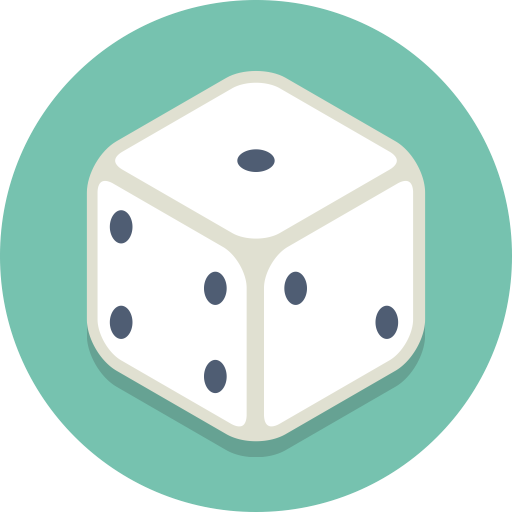The first set of questions to test your quantitative reasoning skills. Improve your ability to solve business problems logically!
10 Min.
10 Fragen
Nicht verfügbar

## Verwandte Cases

### MBB Final Round Case - Smart Education

Themen:
Bewertung:
Our client is SmartBridge, a nonprofit educational institution offering face-to-face tutoring services. The client operates in the US. The mission of SmartBridge is to help as many students as possible to complete studies and prevent that they drop from the school system, in particular in di ... (Ganzen Case öffnen)
19,4 Tsd.
mal gelöst
Schwierig
Bewertung

### Espresso, Whatelse?

Themen:
Bewertung:
Espresso Whatelse is an Italian company that produces coffee and espresso machines since 1908. It is the Italian market leader and has a strong presence overall in Europe. In 2019, Espresso Whatelse has increased its revenues but it has seen declining profit margin. Your client wants to unders ... (Ganzen Case öffnen)
11,9 Tsd.
mal gelöst
Schwierig
Bewertung

### Music Streaming - Market Sizing

Themen:
Bewertung:
Your friend is an aspiring artist and is asking for your consulting advise on a problem that he has been struggling with. Your friend (the artist) is trying to understand how many hours people listen to the number one music streaming platform (the one with the green logo) in total and on average per ... (Ganzen Case öffnen)
4,1 Tsd.
mal gelöst
Anfänger
Bewertung

### Coronavirus Times - COVID-19 Brainteaser

Stil:
Themen:
Bewertung:
You and your family are faced with a challenging set of decisions. Due to coronavirus, your partner has taken a 20% paycut and you are worried you may lose your job. In addition, while daycare is still open, you are worried that sending your two children there will increase the risk of the ... (Ganzen Case öffnen)
3,7 Tsd.
mal gelöst
Anfänger
Bewertung

### Bain 1st Round Case – BlissOttica

Themen:
Bewertung:
Our client is a BlissOttica, an Eyewear Manufacturer that is looking to reach a 10% increase in profits. How would you help our client? ... (Ganzen Case öffnen)
3,3 Tsd.
mal gelöst
Anfänger